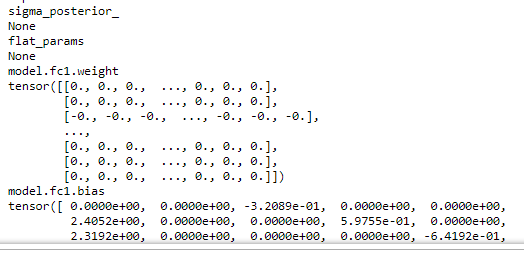# Getting None for parameter gradient

Hi Everyone,

I am new here and started using Pytorch on a project.

I am building my own loss function for a neural network . This is the forward function

``````def forward(self, images, labels):
self.noise = torch.randn(self.d_size).to(self.device) * torch.exp(self.sigma_posterior_)

vector_to_parameters(self.flat_params + self.noise, self.model.parameters())

outputs = self.model(images)

loss = F.cross_entropy(outputs.float(), labels.long())

return loss
``````

The parameters of the loss function are
‘flat_parameters’ :which i am getting by flattening neural network (self.model) weights
“sigma_posterior” : which is a parameter i use for adding noise to network weights.

When trying loss.backward() I am getting the gradient according to the new weights. but I actually want also the gradient according to the previous weights and sigma_posterior.

This is what i am getting for gradient :Thanks for any answer, i will appreciate your help